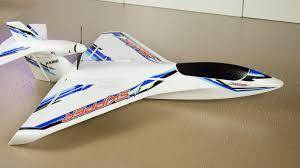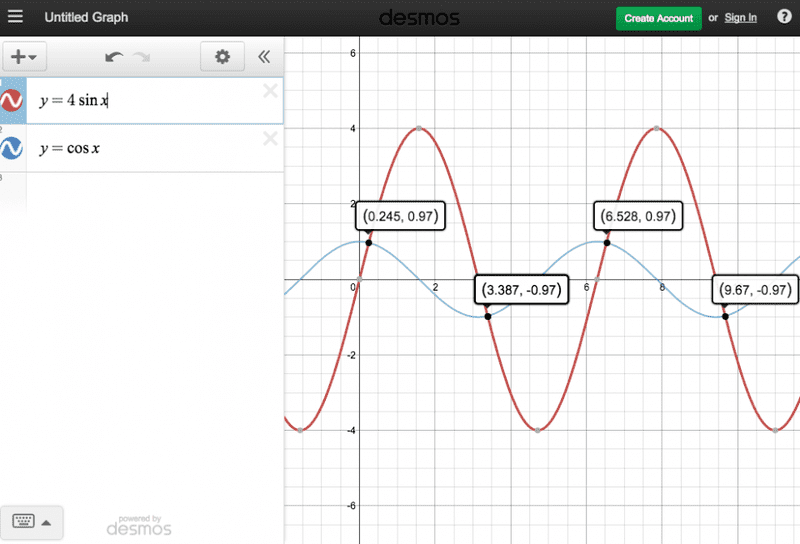# Thrust angle calculation for off-centerline airplane engine

In summary, you would angle the engine down to counteract the pitch-down moment produced by the thrust acting above the CG.

This isn't for any particular project or assignment, but I've tried solving this in the past and haven't figured it out. Say you have an RC seaplane, like the one pictured, with the engine mounted well above and aft the CG. You're going to need to angle it down a little to counteract the pitch-down moment produced by the thrust acting above the CG.Here's what I have so far.
Let's say the motor is 16" behind the CG and 4" above it, and it's generating 5lbs of thrust. To balance the moments, you would set it up like:

(TsinA)y=(TcosA)x

where T=thrust, A=motor angle from x-axis, y=position behind the CG, and x=position above the CG. Plugging in the values:

(5sinA)16=(5cosA)4
...which reduces to:
4sinA=cosA

As far as I know there isn't any way to solve that algebraically. The only way I could think of to solve for A might be graphing the functions f(x)=4sinA and g(x)=cosA and maybe use one of the solutions to find the angle where the two thrust components are equal. Below are the two functions graphed and some of their solutions.So, assuming I'm on the right track, where do I go from here? I initially thought the x-value of each solution would be a possible motor angle, but if you plug that back into the equation above the two sides aren't equal. So how on Earth would I do this?

This isn't for any particular project or assignment, but I've tried solving this in the past and haven't figured it out. Say you have an RC seaplane, like the one pictured, with the engine mounted well above and aft the CG. You're going to need to angle it down a little to counteract the pitch-down moment produced by the thrust acting above the CG.

View attachment 100647

Here's what I have so far.
Let's say the motor is 16" behind the CG and 4" above it, and it's generating 5lbs of thrust. To balance the moments, you would set it up like:

(TsinA)y=(TcosA)x

where T=thrust, A=motor angle from x-axis, y=position behind the CG, and x=position above the CG. Plugging in the values:

(5sinA)16=(5cosA)4
...which reduces to:
4sinA=cosA

As far as I know there isn't any way to solve that algebraically. The only way I could think of to solve for A might be graphing the functions f(x)=4sinA and g(x)=cosA and maybe use one of the solutions to find the angle where the two thrust components are equal. Below are the two functions graphed and some of their solutions.

View attachment 100645

So, assuming I'm on the right track, where do I go from here? I initially thought the x-value of each solution would be a possible motor angle, but if you plug that back into the equation above the two sides aren't equal. So how on Earth would I do this?
As long as you have the same angle A on both sides of the equation, carry the trigonometry forward a little bit:

4 sin (A) = cos (A)

##\frac{sin (A)}{cos (A)} = tan (A) = \frac{1}{4}##

##tan^{-1} (A) = A = tan^{-1} (\frac{1}{4}) ≈ 14° ##

where tan-1 is the arctangent function.

•Okay, that makes sense. Forgot about the sin(A)/cos(A)=tan(A) rule. How did you know that tanA=1/4?

Okay, that makes sense. Forgot about the sin(A)/cos(A)=tan(A) rule. How did you know that tanA=1/4?
You do the algebra on both sides of the equation.

You divide both sides of the equation by cos (A) to get tan (A) on the LHS, which leaves 1 on the RHS.
You then divide both sides of the equation to get just tan (A) on the LHS, which means the RHS is now 1/4.

So, tan (A) = 1/4

•SteamKing said:
You do the algebra on both sides of the equation.

You divide both sides of the equation by cos (A) to get tan (A) on the LHS, which leaves 1 on the RHS.
You then divide both sides of the equation to get just tan (A) on the LHS, which means the RHS is now 1/4.

So, tan (A) = 1/4
Got it. Thanks!

## 1. What is thrust angle calculation and why is it important for off-centerline airplane engines?

Thrust angle calculation is the process of determining the angle at which the thrust of an airplane's engines is directed. This angle is important for off-centerline engines because it affects the overall stability and control of the aircraft during flight.

## 2. How is the thrust angle calculated for off-centerline airplane engines?

The thrust angle is calculated by taking into account the position of the engine relative to the aircraft's centerline, as well as the direction of the engine's thrust vector. This can be done using specialized software or by hand calculations using trigonometry.

## 3. What factors can affect the thrust angle of an off-centerline airplane engine?

Several factors can affect the thrust angle of an off-centerline engine, including the weight and balance of the aircraft, the position of the engine on the wing, and the direction and magnitude of the engine's thrust.

## 4. How does the thrust angle affect the performance of an off-centerline airplane engine?

The thrust angle can significantly impact the performance of an off-centerline engine. If the thrust is not directed along the centerline of the aircraft, it can cause asymmetrical forces and moments that can affect the aircraft's stability, control, and fuel efficiency.

## 5. Can the thrust angle be adjusted for off-centerline airplane engines?

Yes, the thrust angle can be adjusted for off-centerline engines in order to optimize the aircraft's performance and handling. This can be done through engine mount adjustments, or by using thrust vectoring technology to alter the direction of the engine's thrust.